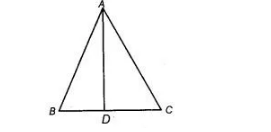# In following figure if A D`
Question:

In following figure if $A D$ is the bisector of $\angle B A C$, then prove that $A B>B D$.Solution:

Given ABC is a triangle such that AD is the bisector of ∠BAC. To prove AB > BD.

Proof Since, AD is the bisector of ∠BAC.